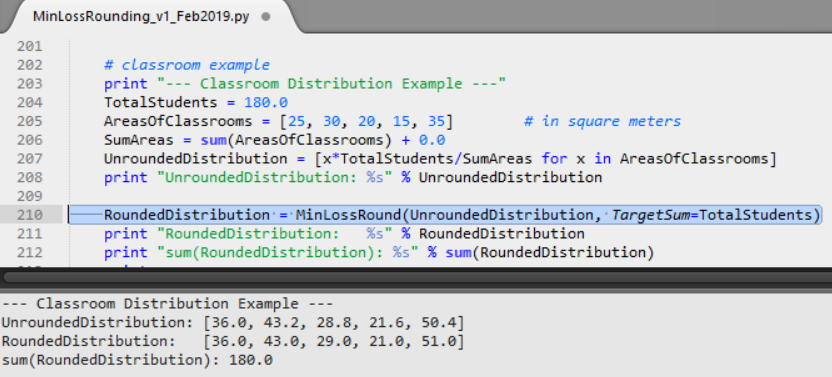# Minimum Total Loss Rounding in Python

Minimum Total Loss Rounding algorithms are quite useful for distribution problems if you need whole numbers after the distribution, as the final result of distribution.```Distribute 180 students to 5 classrooms with given ground areas [25, 30, 20, 15, 35] Sum of all given areas = 25 + 30 + 20 + 15 + 35 = 125 = sum([25, 30, 20, 15, 35]) ```
```areas = [25, 30, 20, 15, 35] d1 = [x*180.0/125.0 for x in areas] ([36.0, 43.2, 28.8, 21.6, 50.4]) ```
In my case, I defined rounding losses in proportionate terms. For example, proportionate round-down loss (deviation) for 43.2 is calculated by the function RoundDownLoss() as follows:``` (43.2 - 43.0) / 43.2 = 0.2 / 43.2 = 0.004629629629629695 ```
And round-up loss (deviation) by the function RoundUpLoss() as follows:``` (44.0 - 43.2) / 43.2 = 0.8 / 43.2 = 0.01851851851851845 ```